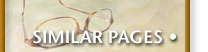## Clojure's Tail Recursion Feature

Lisp-based languages, like mathematical processing, relies on recursion. The quintessential example, discussed ad nauseum, is the factorial function, which is often rendered as it's definition:

``````(defun factorial (n)
(if (< n 2) 1
(* n (factorial (- n 1)))))

(factorial 5)  ;; Returns 120
``````

The problem with this approach is large calculations that "blow" the stack. Some systems, catch the exception and move the entire world over to heap.

The traditional solution is to use tail recursion. If the last operation in a function is a recursive call to that function, then it is easy for the language to treat the operation as a loop.

``````(defun factorial (n)
(defun inner-factorial (cnt acc)
(if (< cnt 2) acc
(inner-factorial (- cnt 1) (* acc cnt))))
(inner-factorial n 1))

(factorial 5)
``````

Notice the hallmarks of this approach:

1. An inner function that does the work
2. An accumulator that holds the current calculation
3. The accumulator is returned when the break-out condition is reach.

While Clojure is a Lisp-based language, it also runs on top of a host virtual machine (usually the JVM), and currently the JVM does not support tail recursion.

The solution was to create the `loop` -- `recur` construct. Here is a rendering of the previous code in Clojure:

``````(defn factorial [n]
(loop [cnt n
acc 1]
(if (< cnt 2) acc
(recur (dec cnt) (* cnt acc)))))

(factorial 5)
``````

At first, I didn't care much for the special consideration for dealing with tail recursion that just happens in other Lisp languages, however, I've changed my mind for the following reasons:

First, we often have to have an accumulator that we hide inside an inner function or lambda, the `loop` simply replaces what we normally do anyway.

Second, while you may want tail recursion, you may not be sure you are getting tail recursion. The Clojure approach ensures that `recur` is the last call in the function, and throws an error if not.

What appeared to me to be a kludge at first, has become a feature to me.

Tell others about this article: